Instructions

For the following questions answer them individually

Question 1

# At time t = 0, terminal A in the circuit shown in the figure is connected to B by a keyand an alternating current $$I(t) = I_0 \cos^fo (\omega t)$$, with $$I_0 = 1 A$$ and $$\omega = 500 rad s^{-1}$$ starts flowing in it with the intial direction shown in the figure. At $$t = \frac{7 \pi}{6 \omega}$$, the key is switched from B to D. Now onwards only A and D are connected. A total charge Q flows from the battery to charge the capacitor fully. If $$C = 20 \mu F, R = 10 Ω$$ and the battery is ideal with emf of 50V, identify the correct statement (s).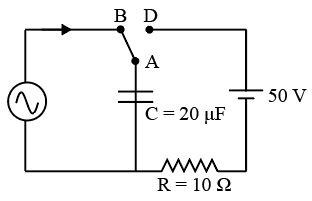Question 2

# A light source, which emits two wavelengths $$\lambda_1 = 400 nm$$ and $$\lambda_2 = 600 nm$$, is used in a Young’s double slit experiment. If recorded fringe widths for $$\lambda_1$$ and $$\lambda_2$$ are $$\beta_1$$ and $$\beta_2$$ number of fringes for them within a distance yon one side of the central maximum are $$m_1$$ and $$m_2$$, respectively, thenQuestion 3

# One end of a taut string of length 3m along the x axis is fixed at x = 0.The speed of the waves in the string is $$100 ms^{-1}$$. The other end of the string is vibrating in the y direction so that stationary waves are set up in the string. The possible waveform(s) of these stationary waves is(are)Question 4

# A parallel plate capacitor has a dielectric slab of dielectric constant K between its plates that covers $$\frac{1}{3}$$ of the area of its plates, as shown in the figure. The total capacitance of the capacitor is C while that of the portion with dielectric in between is $$C_1$$. When the capacitor is charged, the plate area covered by the dielectric gets charge $$Q_1$$ and the rest of the area gets charge $$Q_2$$. The electric field in the dielectric is $$E_1$$ and that in the other portion is $$E_2$$. Choose the correct option/options, ignoring edge effects.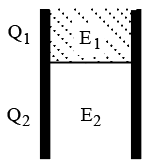Question 5

# Let $$E_1 (r), E_2 (r)$$ and $$E_3 (r)$$ be the respective electric fields at a distance r from a point charge Q, an infinitely long wire with constant linear charge density $$\lambda$$, and an infinite plane with uniform surface charge density $$\sigma$$. If $$E_1 (r_0) = E_2 (r_0) = E_3 (r_0)$$ at a given distance $$r_0$$, thenQuestion 6

# A student is performing an experiment using a resonance column and a tuning fork of frequency $$244s^{−1}$$. He is told that the air in the tube has been replaced by another gas (assume that the column remains filled with the gas). If the minimum height at which resonance occurs is $$(0.350 \pm 0.005) m,$$ the gas in the tube is(Useful information: $$\sqrt {167 RT} = 640 J^{\frac{1}{2}} mole^{\frac{-1}{2}} ;\sqrt {140 RT} = 590 J^{\frac{1}{2}} mole^{\frac{-1}{2}}$$.The molar masses Min grams are given in the options. Take the values of $$\sqrt{\frac{10}{M}}$$ for each gas as given there.)Question 7

# Heater of an electric kettle is made of a wire of length L and diameter d. It takes 4 minutes to raise the temperature of 0.5kg water by 40K. This heater is replaced by a new heater having two wires of the same material, each of length L and diameter 2d.The way these wires are connected is given in the options. How much time in minutes will it take to raise the temperature of the same amount of water by 40 K?Question 8

# In the figure, a ladder of mass m is shown leaning against a wall. It is in static equilibrium making an angle $$\theta$$ with the horizontal floor. The coefficient of friction between the wall and the ladder is $$\mu_1$$ and that between the floor and the ladder is $$\mu_2$$. The normal reaction of the wall on the ladder is $$N_1$$ and that of the floor is $$N_2$$. If the ladder is about to slip, then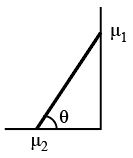Question 9

# A transparent thin film of uniform thickness and refractive index $$n_1 = 1.4$$ is coated on the convex spherical surface of radius Rat one end of a long solid glass cylinder of refractive index $$n_2 = 1.5,$$ as shown in the figure. Rays of light parallel to the axis of the cylinder traversing through the film from air to glass get focused at distance $$f_1$$ from the film, while rays of light traversing from glass to air get focused at distance $$f_2$$ from the film. Then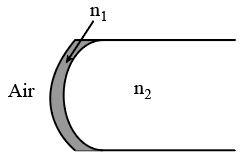Question 10

# Two ideal batteries of  $$emfV_1$$ and $$V_2$$ and three resistances $$R_1, R_2$$ and $$R_3$$ are connected as shown in the figure. The current in resistance $$R_2$$ would be zero if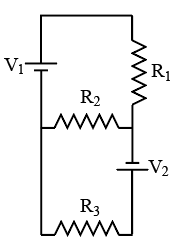OR